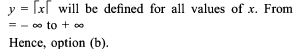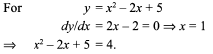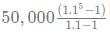Courses

# Test: Functions- 3

## 15 Questions MCQ Test Quantitative Aptitude for SSC CGL | Test: Functions- 3

Description
This mock test of Test: Functions- 3 for Quant helps you for every Quant entrance exam. This contains 15 Multiple Choice Questions for Quant Test: Functions- 3 (mcq) to study with solutions a complete question bank. The solved questions answers in this Test: Functions- 3 quiz give you a good mix of easy questions and tough questions. Quant students definitely take this Test: Functions- 3 exercise for a better result in the exam. You can find other Test: Functions- 3 extra questions, long questions & short questions for Quant on EduRev as well by searching above.
QUESTION: 1

### Find the domain of the definition of the function y = lxl

Solution:QUESTION: 2

### If f(x) is an even function, then the graph y = f(x) will be symmetrical about

Solution:

y — axis by definition.

QUESTION: 3

### In the previous question, what will be the minimum value of the function?

Solution:

Required value = (-5)2 + 10(-5) + 11 = 25-50 + 11 =-14.

QUESTION: 4

Find the minimum value of the function fix) = log2 (x2 - 2x + 5).

Solution:

The minimum value of the function would occur at the minimum value of (x2 - 2x + 5) as this quadratic function has imaginary roots.Thus, minimum value of the argument of the log is 4. So minimum value of the function is log2 4 = 2.

QUESTION: 5

If f(x) is an even function, then the graph y = f(x) will be symmetrical about

Solution:

y – axis by definition.

QUESTION: 6

Which of the following is an even function?

Solution:

x–8 is even since f(x) = f(–x) in this case.

QUESTION: 7

For what value of x, x2 + 10x + 11 will give the minimum value?

Solution:

dy/dx = 2x + 10 = 0 fi x = –5.

QUESTION: 8

Find the maximum value of the function 1/(x2 – 3x + 2).

Solution:

Since the denominator x2 – 3x + 2 has real roots, the maximum value would be infinity.

QUESTION: 9

Read the instructions below and solve.
f(x) = f(x – 2) – f(x – 1), x is a natural number
f(1) = 0, f(2) = 1

The value of f(8) is

Solution:

f(1) = 0, f(2) = 1,
f(3) = f(1) – f(2) = –1
f(4) = f(2) – f(3) = 2
f(5) = f(3) – f(4) = –3
f(6) = f(4) – f(5) = 5
f(7) = f(5) – f(6) = –8
f(8) = f(6) – f(7) = 13
f(9) = f(7) – f(8) = –21

13

QUESTION: 10

Read the instructions below and solve.
f(x) = f(x – 2) – f(x – 1), x is a natural number
f(1) = 0, f(2) = 1

What will be the domain of the definition of the function f(x) = 8–xC 5–x for positive values of x?

Solution:

f(1) = 0, f(2) = 1,
f(3) = f(1) – f(2) = –1
f(4) = f(2) – f(3) = 2
f(5) = f(3) – f(4) = –3
f(6) = f(4) – f(5) = 5
f(7) = f(5) – f(6) = –8
f(8) = f(6) – f(7) = 13
f(9) = f(7) – f(8) = –21

For any nCr, n should be positive and r ≥ 0.
Thus, for positive x, 5 – x ≥ 0
fi x = 1, 2, 3, 4, 5.

QUESTION: 11

Ajesh saves Rs 50,000 every year and deposits the money in a bank at compound interest of 10%(compunded annually).What would be his total saving at the end of the 5th year?

Solution:

At the end of the 1st year, he will get Rs 50000, it will give him interest for 4 years compounded annually
Hence at the end of 5 years, this amount will become 50000(1.1)4
Similarly, the amount deposited in the 2nd year will give interest for 3 years. Hence it will become 50000(1.1)3
Similarly, we can calculate for the remaining years.
The total saving at the end of the 5th year would be a GP, given by
Net saving = 50000(1.1)+  50000(1.1)3  ..... 50000
Thus net saving ==  Rs 3,05,255

QUESTION: 12

Define the following functions:
(a) (a M b) = a – b (b) (a D b) = a + b
(c) (a H b) = (ab) (d) (a P b) = a/b

Q.

Which of the following functions will represent a2 – b2?

Solution:

Option a = (a – b) (a + b) = a2 – b2

QUESTION: 13

Define the following functions:
(a) (a M b) = a – b (b) (a D b) = a + b
(c) (a H b) = (ab) (d) (a P b) = a/b

Q.

What is the value of (3M4H2D4P8M2)?

Solution:

3 – 4 × 2 + 4/8 – 2 = 3 – 8 + 0.5 – 2 = – 6.5
(using BODMAS rule)

QUESTION: 14

Define the following functions:
(a) (a M b) = a – b (b) (a D b) = a + b
(c) (a H b) = (ab) (d) (a P b) = a/b

Q.

Which of the four functions defined has the minimum value?

Solution:

The minimum would depend on the values of a and b. Thus, cannot be determined.

QUESTION: 15

If 0 < a <1 and 0 < b < 1 and if a < b, which of the following expressions will have the highestvalue?

Solution:

Again (a + b) or a/b can both be greater than each other depending on the values we take for a and b.
E.g. for a = 0.9 and b = 0.91, a + b > a/b.
For a = 0.1 and b = 0.11, a + b < a/b